## RD Sharma Class 8 Solutions Chapter 2 Powers MCQS

These Solutions are part of RD Sharma Class 8 Solutions. Here we have given RD Sharma Class 8 Solutions Chapter 2 Powers MCQS

Other Exercises

Choose the correct alternative in each of the following :

Question 1.
Square of $$\left( \frac { -2 }{ 3 } \right)$$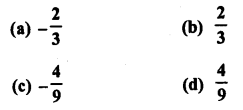Solution:Question 2.
Cube of $$\frac { -1 }{ 2 }$$ isSolution:Question 3.
Which of the following is not equal to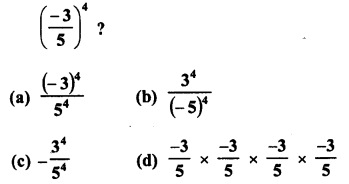Solution: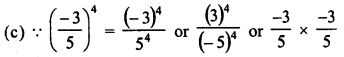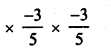Question 4.
Which of the following in not reciprocal ofSolution:Question 5.
Which of the following numbers is not equal to $$\frac { -8 }{ 27 }$$ ?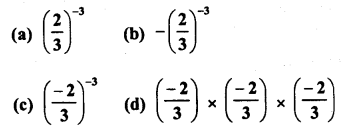Solution: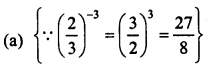Question 6.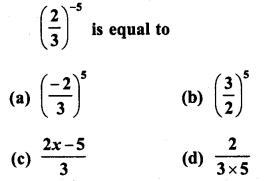Solution: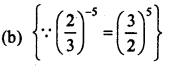Question 7.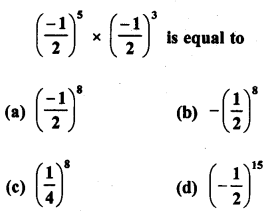Solution: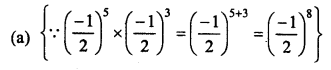Question 8.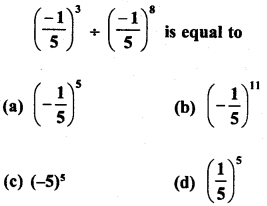Solution:Question 9.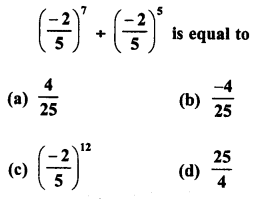Solution:Question 10.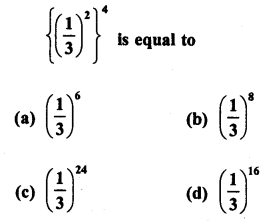Solution:Question 11.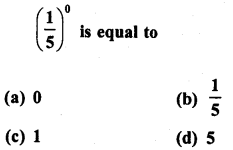Solution:Question 12.Solution:Question 13.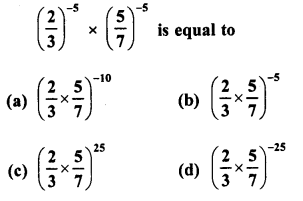Solution: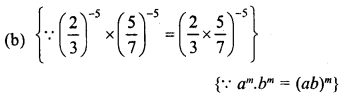Question 14.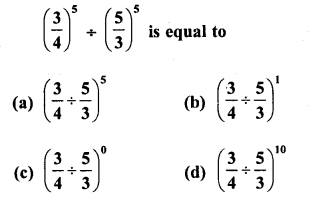Solution: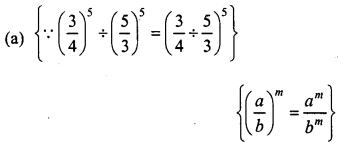Question 15.
For any two non-zero rational numbers a and b,a4+b4 is equal to
(a) (a + b)1
(b) (a + b)0
(c) (a + b)4
(d) (a + b)8
Solution:
(c) {∵ a4 + b4 = (a + b)4}

Question 16.
For any two rational numbers a and b, a5 x b5 is equal to
(a) (a x b)0
(b) (a x b)10

(c) (a x b)5
(d) (a x b)25

Solution:
(c) {∵ a5 x b5 = (a x b)5}

Question 17.
For a non-zero rational number a, a7 + a12 is equal to
(a) a5
(b) a-19

(c) a-5
(d) a19

Solution:
(c) {a5 a12 = a7-12 =a-5}

Question 18.
For a non-zero rational number a, (a3)-2 is equal to
(a) a6
(b) a-6
(c) a-9
(d) a1

Solution:
(b) {(a3)-2 = a3 x (-2)= a6}

Hope given RD Sharma Class 8 Solutions Chapter 2 Powers MCQS are helpful to complete your math homework.

If you have any doubts, please comment below. Learn Insta try to provide online math tutoring for you.